# Non parametric noise estimation

In order to provide a fully automatic denoising algorithm, we have developed an automatic noise estimation method that relies on the non-parametric detection of homogeneous areas. First, the homogeneous regions of the image are detected by computing Kendall’s rank correlation coefficient . Computed on neighboring pixel sequences, it indicates the dependancy between neighbors, hence reflects the presence of structure inside an image block. This test is non-parametric, so the performance of the detection is independant of the noise statistical distribution. Once the homogeneous areas are detected, the noise level function, i.e., the function of the noise variance with respect to the image intensities, is estimated as a second order polynomial minimizing the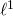error on the statistics of these regions.

Matlab implementation of the noise estimation algorithm

Related papers:

– C. Sutour, C.-A. Deledalle et J.-F. Aujol. Estimation of the noise level function based on a non-parametric detection of homogeneous image regions. Submitted to Siam Journal on Imaging Sciences, 2015.

– C. Sutour, C.-A. Deledalle et J.-F. Aujol. Estimation du niveau de bruit par la détection non paramétrique de zones homogènes. Submitted to Gretsi, 2015.

## References

 Buades, A., Coll, B., and Morel, J.-M. (2005). A review of image denoising algorithms, with a new one. Multiscale Modeling and Simulation, 4(2): 490–530.

The denoising algorithm that has been developed is based on an adaptive regularization of the NL-means . The proposed model is the following:

(1)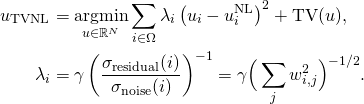where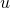is the solution obtained with the NL-means algorithm, TV refers to the total variation of the image and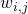is the weight that measures the similarity between the patch of indexand the patch of indexin the NL-means algorithm. The ratioreflects the noise variance reduction performed by the NL-means. This formulation allows locally adaptive regularization of the NL-means solution, thanks to a confidence index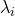that reflects the quality of the denoising performed by the NL-means.

This model can be adapted to the different noise statistics belonging to the exponential family (Gaussian, Poisson, multiplicative…). It can also be adapted to video denoising thanks to the use of 3D patches combined to a spatio-temporal TV regularization.

Matlab implementation of RNL

Results of video denoising with R-NL and comparisons

Related papers:
1. C. Sutour, C.-A. Deledalle et J.-F. Aujol. Adaptive regularization of the NL-means : Application to image and video denoising. IEEE Transactions on image processing, vol. 23(8) : 3506-3521, 2014.

2. C. Sutour, J.-F. Aujol, C.-A. Deledalle et J.-P. Domenger. Adaptive regularization of the NL-means for video denoising. International Conference on Image Processing (ICIP), pages 2704–2708. IEEE, 2014.

3. C. Sutour, J.-F. Aujol et C.-A. Deledalle. TV-NL : Une coopération entre les NL-means et les méthodes variationnelles. Gretsi, 2013.

## References

 Buades, A., Coll, B., and Morel, J.-M. (2005). A review of image denoising algorithms, with a new one. Multiscale Modeling and Simulation, 4(2): 490–530.

# Edge based multi-modal registration

In order to perform multi-modal fusion between images obtained from a light intensifier (LI) and IR (infra-red) images, hence providing multi-sources information to the pilot, it is necessary to perform registration between the two modalities. They reflect different properties of the scene, so the selected approach is based on the geometric information and the edges of each image.

The goal is to find the transformationthat transfers the IL image into the frame of reference of the IR image by minimizing the following energy:

(1)This metric is based on the edges of each image, and it only takes into account the edges that occur in both modalitites, which makes it insensitive to outliers.
Thanks to a gradient descent based optimization scheme, it is possible to quickly evaluate to optimal transformation between the two modalities.

Registration results on real data

Related papers:
C. Sutour, J.-F. Aujol, C.-A. Deledalle et B.D. De-Senneville. Edge-based multi-modal registration and application for night vision devices. Journal of Mathematical Imaging and Vision, pages 1–20, 2015.